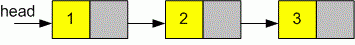Competitions

# LinkedList Length

Given a linked list, find its length. The length of a linked list is the number of its elements.

Definition of a single linked list:

// Java
class ListNode {
int val;
ListNode next;
ListNode(int x) {
val = x;
next = null;
}
}

// C++
class ListNode
{
public:
int val;
ListNode *next;
ListNode(int x) : val(x), next(NULL) {}
};

// C
struct ListNode
{
int val;
struct ListNode *next;
};


Implement function length to find the length of linked list.

// Java
int length(ListNode head)

// C, C++
int length(ListNode *head)


#### ExampleThe length of a linked list is 3.

Time limit 1 second
Memory limit 128 MiB
Author Mykhailo Medvediev